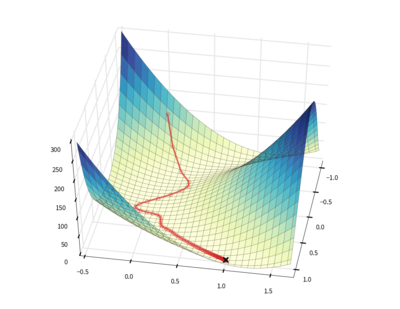The downhill package provides algorithms for minimizing scalar loss functions that are defined using Theano.

Several optimization algorithms are included:

All algorithms permit the use of regular or Nesterov-style momentum as well.

The source code for downhill lives at http://github.com/lmjohns3/downhill, the documentation lives at http://downhill.readthedocs.org, and announcements and discussion happen on the mailing list.

# Quick Start: Matrix Factorization¶

Let’s say you want to compute a sparse, low-rank approximation for some 1000-dimensional data that you have lying around. You can represent a batch of $$m$$ of data points $$X \in \mathbb{R}^{m \times 1000}$$ as the product of a sparse coefficient matrix $$U \in \mathbb{R}^{m \times k}$$ and a low-rank basis matrix $$V \in \mathbb{R}^{k \times 1000}$$. You might represent the loss as

$\mathcal{L} = \| X - UV \|_2^2 + \alpha \| U \|_1 + \beta \| V \|_2$

where the first term represents the approximation error, the second represents the sparsity of the representation, and the third prevents the basis vectors from growing too large.

This is pretty straightforward to model using Theano. Once you set up the appropriate variables and an expression for the loss, you can optimize the loss with respect to the variables using a single call to downhill.minimize():

import climate
import downhill
import numpy as np
import theano
import theano.tensor as TT

climate.enable_default_logging()

def rand(a, b): return np.random.randn(a, b).astype('f')

A, B, K = 20, 5, 3

# Set up a matrix factorization problem to optimize.
u = theano.shared(rand(A, K), name='u')
v = theano.shared(rand(K, B), name='v')
e = TT.sqr(TT.matrix() - TT.dot(u, v))

# Minimize the regularized loss with respect to a data matrix.
y = np.dot(rand(A, K), rand(K, B)) + rand(A, B)

downhill.minimize(
loss=e.mean() + abs(u).mean() + (v * v).mean(),
train=[y],
patience=0,
batch_size=A,                 # Process y as a single batch.
max_gradient_norm=1,          # Prevent gradient explosion!
learning_rate=0.1,
monitors=(('err', e.mean()),  # Monitor during optimization.
('|u|<0.1', (abs(u) < 0.1).mean()),
('|v|<0.1', (abs(v) < 0.1).mean())),
monitor_gradients=True)

# Print out the optimized coefficients u and basis v.
print('u =', u.get_value())
print('v =', v.get_value())